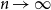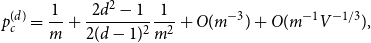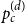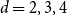Home

# Expansion of Percolation Critical Points for Hamming Graphs

## Abstract

The Hamming graph H(d, n) is the Cartesian product of d complete graphs on n vertices. Let${m=d(n-1)}$ be the degree and$V = n^d$ be the number of vertices of H(d, n). Let$p_c^{(d)}$ be the critical point for bond percolation on H(d, n). We show that, for$d \in \mathbb{N}$ fixed and$n \to \infty$ ,$$p_c^{(d)} = {1 \over m} + {{2{d^2} - 1} \over {2{{(d - 1)}^2}}}{1 \over {{m^2}}} + O({m^{ - 3}}) + O({m^{ - 1}}{V^{ - 1/3}}),$$
which extends the asymptotics found in  by one order. The term$O(m^{-1}V^{-1/3})$ is the width of the critical window. For$d=4,5,6$ we have$m^{-3} = O(m^{-1}V^{-1/3})$ , and so the above formula represents the full asymptotic expansion of$p_c^{(d)}$ . In  we show that this formula is a crucial ingredient in the study of critical bond percolation on H(d, n) for$d=2,3,4$ . The proof uses a lace expansion for the upper bound and a novel comparison with a branching random walk for the lower bound. The proof of the lower bound also yields a refined asymptotics for the susceptibility of a subcritical Erdös–Rényi random graph.

## Corresponding author

*Corresponding author. Email: Lorenzo.Federico@warwick.ac.uk

Hide All

# Expansion of Percolation Critical Points for Hamming Graphs

## Metrics

### Full text viewsFull text views reflects the number of PDF downloads, PDFs sent to Google Drive, Dropbox and Kindle and HTML full text views.

Total number of HTML views: 0
Total number of PDF views: 0 *Loading metrics...

### Abstract viewsAbstract views reflect the number of visits to the article landing page.

Total abstract views: 0 *Loading metrics...

* Views captured on Cambridge Core between <date>. This data will be updated every 24 hours.

Usage data cannot currently be displayed